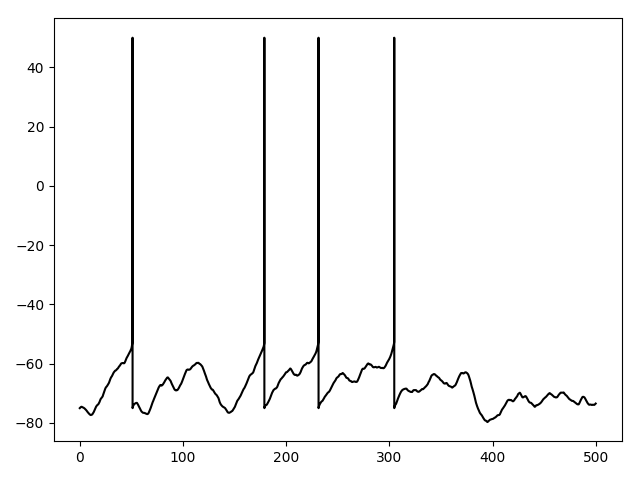# Example: Fig5A¶

Note

You can launch an interactive, editable version of this example without installing any local files using the Binder service (although note that at some times this may be slow or fail to open):Brette R (2013). Sharpness of spike initiation in neurons explained by compartmentalization. PLoS Comp Biol, doi: 10.1371/journal.pcbi.1003338.

Fig. 5A. Voltage trace for current injection, with an additional reset when a spike is produced.

Trick: to reset the entire neuron, we use a set of synapses from the spike initiation compartment where the threshold condition applies to all compartments, and the reset operation (v = EL) is applied there every time a spike is produced.

from brian2 import *
from params import *

defaultclock.dt = 0.025*ms
duration = 500*ms

# Morphology
morpho = Soma(50*um)  # chosen for a target Rm
morpho.axon = Cylinder(diameter=1*um, length=300*um, n=300)

# Input
taux = 5*ms
sigmax = 12*mV
xx0 = 7*mV

compartment = 40

# Channels
eqs = '''
Im = gL * (EL - v) + gNa * m * (ENa - v) + gLx * (xx0 + xx) : amp/meter**2
dm/dt = (minf - m) / taum : 1  # simplified Na channel
minf = 1 / (1 + exp((va - v) / ka)) : 1
gNa : siemens/meter**2
gLx : siemens/meter**2
dxx/dt = -xx / taux + sigmax * (2 / taux)**.5 *xi : volt
'''

neuron = SpatialNeuron(morphology=morpho, model=eqs, Cm=Cm, Ri=Ri,
threshold='m>0.5', threshold_location=compartment,
refractory=5*ms)
neuron.v = EL
neuron.gLx = gL
neuron.gNa[compartment] = gNa_0 / neuron.area[compartment]

# Reset the entire neuron when there is a spike
reset = Synapses(neuron, neuron, on_pre='v = EL')
reset.connect('i == compartment')  # Connects the spike initiation compartment to all compartments

# Monitors
S = SpikeMonitor(neuron)
M = StateMonitor(neuron, 'v', record=0)
run(duration, report='text')